Li, B., Zhang, Z., Li, W., and Peng, X. (2015). "Model for tangential tensioning stress in the edge of circular saw blades tensioned by multi-spot pressure," BioRes. 10(2), 3798-3810.

#### Abstract

In this study, a mathematical model of tangential tensioning stress in the edge of a circular saw blade tensioned by multi-spot pressure was established by theoretical analysis for the quality control of circular saw blades. The multi-spot pressure tensioning process was assumed to include three mechanical processes: the one-spot pressure process; the process of elastic deformation of a disk with a through-hole subjected to uniform, radial compressive stress; and the stress superposition process. The tangential tensioning stress on the edge of the circular saw blade could be accurately predicted by the established mathematical model. The model for the tangential tensioning stress on the edge of the circular saw blade tensioned by multi-spot pressure was shown to be correct by measured values.

Model for Tangential Tensioning Stress in the Edge of Circular Saw Blades Tensioned by Multi-Spot Pressure

Bo Li,* Zhankuan Zhang, Weiguang Li, and Xiaorui Peng

In this study, a mathematical model of tangential tensioning stress in the edge of a circular saw blade tensioned by multi-spot pressure was established by theoretical analysis for the quality control of circular saw blades. The multi-spot pressure tensioning process was assumed to include three mechanical processes: the one-spot pressure process; the process of elastic deformation of a disk with a through-hole subjected to uniform, radial compressive stress; and the stress superposition process. The tangential tensioning stress on the edge of the circular saw blade could be accurately predicted by the established mathematical model. The model for the tangential tensioning stress on the edge of the circular saw blade tensioned by multi-spot pressure was shown to be correct by measured values.

Keywords: Circular saw blade; Multi-spot pressure tensioning; Finite element method

Contact information: Research Institute of Wood Industry, Chinese Academy of Forestry, Beijing 100091, China; *Corresponding author: libohongxing@sina.com

INTRODUCTION

With rapid economic development and improving environmental awareness, wood processing enterprises are increasingly requiring highly-effective, sustainable wood use. In the field of wood products manufacturing, the circular saw blade is an indispensable tool. Wood processing enterprises have placed greater demand on its cutting precision and material saving ability.

When a circular saw blade is cutting a work piece, tangential and radial tensile stresses are produced because of the centrifugal force generated by the high rotational speed of the blade. Thermal stress is also produced because the temperature at the edge of the blade is higher than in other regions. These two stresses cause large tangential compressive stress in the edge of the circular saw blade, causing buckling deformation that reduces cutting precision, increases kerf loss, and shortens the saw life.

Tensioning processes such as hammering, rolling, and heating are applied to avoid deformation. Tangential tensile stress in the edge of the circular saw blade is produced during the tensioning process to compensate for the tangential compressive stress, which improves the stability of the blade. Therefore, the tangential tensioning stress in the edge of the circular saw blade is the key factor determining its stability.

The distribution of tangential tensioning stress in the circular saw blade after roll tensioning is not ideal because it decreases gradually with the radius from the loop of the tensioned region to the outside (Szymani and Mote 1979). The tangential tensioning stresses in circular saw blades tensioned by three levels of hammering were measured by an X-ray stress meter (Umetsu 1989; Umetsu et al. 1994). The tangential tensioning stress outside the hammered region increases with radius, so that the maximum tensile stress is at the edge of the blade. This indicates that the tangential tensioning stress produced by hammering is more beneficial than that produced by roll tensioning. However, hammer tensioning has limitations because its reproducibility and controllability are poor, and it depends heavily on the experience and skill of the saw repair worker.

The multi-spot pressure tensioning process is one kind of tensioning method. Fixed areas of the circular saw blade are pressed by spherical or cylindrical surfaces during the multi-spot pressure tensioning process. This approach is conducive to automatic operation because the location and pressure can be accurately controlled.

At present, studies of the tensioning processes of circular saw blades have mainly focused on the effect of tensioning on the dynamic stability of the blades (Carlin 1975; Szymani and Mote 1974; Schajer and Mote 1983; Schajer and Mote 1984; Schajer and Kishimoto 1996; Stakhiev 2004; Ishihara et al.2010; Cristóvão et al. 2012; Ishihara et al. 2012). Only a few studies have examined the generation of tensioning stress during the roll tensioning process. A theoretical model of the roll tensioning process was established by Szymani (Szymani and Mote 1979). The tensioning stresses obtained by the model were generally in agreement with the experimentally determined tensioning stresses (Szymani and Mote 1979). A model of the roll tensioning process was established by Nicoletti based on the finite element method, and the tensioning stresses were determined (Nicoletti et al. 1996). A FEM simulation model for roll tensioning of circular saw blades, which allowed the investigation of various roll tensioning parameters, was developed by Heisel et al. (2014). To date, there has been little theoretical research about the generation of tensioning stress during the multi-spot pressure tensioning process. According to the multi-spot pressure tensioning process, there is no mathematical model to describe the relationship between process parameters and tangential tensioning stress in the edge of circular saw blades. Without the mathematical model, the effect of the multi-spot pressure tensioning process cannot be predicted effectively.

The tangential tensioning stress in the edge of circular saw blades tensioned by multi-spot pressure is the key factor that describes the tensioning effect and determines the quality of the saw blade. The objective of this study was to establish a mathematical model for the tangential tensioning stress in the edge of circular saw blades tensioned by multi-spot pressure based on the theory of elastic mechanics and finite element method.

EXPERIMENTAL

Materials

The parameters of the circular saw blade are shown below. The material was 65 Mn; the hardness was HRC42; the diameter was 356 mm; the thickness was 2.2 mm; and the diameter of the hole was 30 mm. The yield strength of the circular saw blade was 430 MPa. Its elastic modulus and Poisson ratio were 210 GPa and 0.3, respectively. The parameters of the spherical pressure head are shown below. Its hardness was HRC60; its radius was 70 mm; and the loading force was 45.6 kN. The distribution of spots is shown in Fig. 1.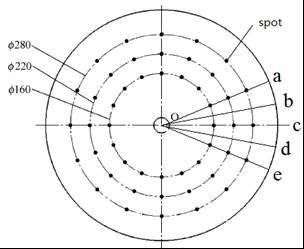Fig. 1. Distribution of spots on circular saw blade

Methods

Tangential tensioning stress measurement

As shown in Fig. 1, the tangential tensioning stresses of regions near the fixed points a, b, c, d, and e were measured by an X-ray stress meter, with reference to Umetsu (Umetsu 1989; Umetsu et al. 1994). To improve test precision, the tangential tensioning stress of each point was tested twice with the X-ray stress meter, as shown in Fig. 2. The average value of the results at each point was reported as the final tangential tensioning stress.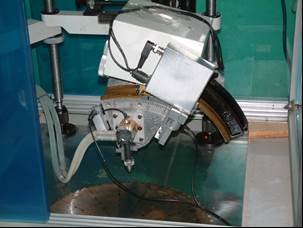Fig. 2. X-ray stress meter

Simplified mechanical model for pressure tensioning process of circular saw blade

According to the multi-spot pressure tensioning process of circular saw blades, the following assumptions were made in creating the mechanical model. First, the shape of the local, plastic deformation region was assumed to be circular, as seen from the surface, because the shape of the head was spherical and the metal in the plastic deformation region flow around evenly. Second, the non-uniform distribution of stress and strain in the thickness direction of the circular saw blade was assumed to have very little effect. Third, the local plastic deformation behaviors of different pressure positions were assumed to be exactly the same. The effect between the local plastic deformation behaviors of different pressure positions was assumed to be very little. An axial symmetry model was built for the one-spot pressure process considering its axial symmetry.

Fourth, the local plastic deformation region of the circular saw blade was replaced by an axial through-hole. The inner wall of the through-hole was subjected to uniform, radial compressive stress. The stress field obtained by elastic mechanics was approximately equivalent to the tensioning stress field of the circular saw blade tensioned by multi-spot pressure.

The mechanical model of the multi-spot pressure tensioning process of the circular saw blade is shown in Fig. 3 and is based on the above assumptions. The radius, m, of the axial through-hole was the same as the radius of the local plastic deformation region. The uniform radial compressive stress, qa, applied to the inner wall of the through-hole was equal to the average radial compressive stress at the boundary of the local plastic deformation region.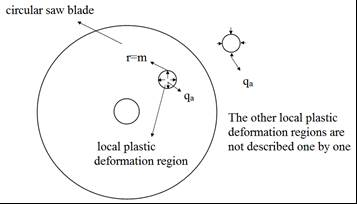Fig. 3. Mechanical model of the multi-spot pressure tensioning process of circular saw blades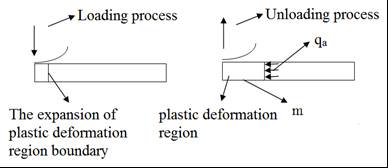Fig. 4. Mechanical model of the one-spot pressure process

Establishment and numerical analysis of one spot pressure process of circular saw blade

An axially symmetrical model was established to simulate the one-spot pressure process, as shown in Fig. 4; m and qa can be calculated using this mechanical model. A mathematical model describing the relationship between the radius of the spherical head, Rt, the loading force, Pm, and qa could be built via the finite element method (FEM).

The geometric model and the model after meshing are shown in Figs. 5 and 6, respectively. The material model of the circular saw blade was set as a linear strengthening elastic-plastic model. Its strain hardening rate was 1000 MPa. A vertical displacement constraint was applied to the axial center plane. An axial symmetry constraint was applied to the axis of the saw blade. The element CAX4R was chosen for the circular saw blade. The number of elements was increased within the contact area between the circular saw blade and the spherical head. The spherical head was modeled as an analytical rigid body. The loading force was applied to the spherical pressure head.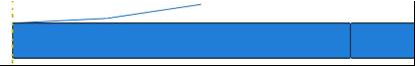Fig. 5. Schematic diagram of geometric model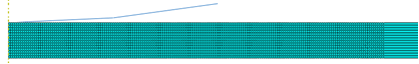Fig. 6. Schematic diagram of model after meshing

Rt was 35, 40, 45, or 50 mm, and P was 60, 70, 80, or 90 kN. As shown in Fig. 7, m increased linearly with P, because increases in P promoted the expansion of the plastic zone. The curves expressing m as a function of P shifted downward with increasing Rt because the average contact stress decreased with Rt. Based on the linear fitting method, m could be expressed as follows (Eq. 1),

m = 0.112(P – 35) + 6.96 – 0.016(Rt – 60) (1)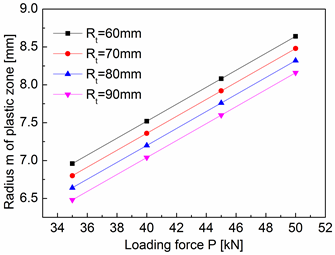As shown in Fig. 8, qa was approximately 212.5 MPa because the metal in the junction between the elastic and plastic deformation zones had the same stress state.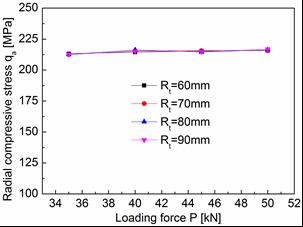Establishment and numerical analysis of the circular saw blade with a through-hole subjected to uniform radial compressive stress

As shown in Fig. 9, the circular saw blade had a through-hole whose inner wall was subjected to uniform radial compressive stress qa. The origin of the polar coordinates was located in the center of the through-hole. The tangential stress σθt of the blade edge with respect to the polar coordinate system was calculated to establish the mathematical relationship between σθt and θti.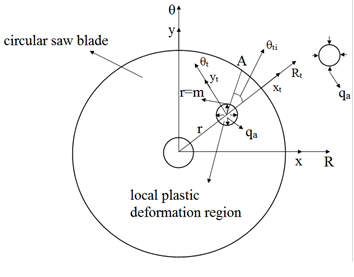Fig. 9. Mechanical model of circular saw blade with a through-hole subjected to uniform radial compressive stress

A plane stress finite element model was built considering the symmetry of the model, as shown in Figs. 10 and 11. A vertical displacement constraint was applied to the plane of symmetry. A coupling constraint was applied to the inner wall of the center hole of the circular saw blade. The constraint point was the center of the circular saw blade and all of its degrees of freedom were constrained. The uniform radial compressive stress applied to the inner wall of the through-hole was 212.5 MPa. The element CPS4R was chosen for the circular saw blade. The number of elements was increased near the through-hole to improve calculation precision. The total number of elements was 5689.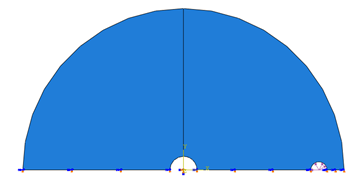Fig. 10. Schematic diagram of geometric model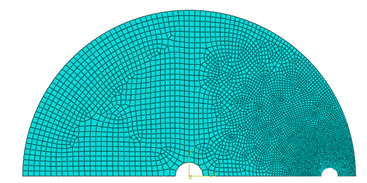Fig. 11. Schematic diagram of model after meshing

As shown in Fig. 12, the relationship between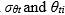, was expressed as follows (Eq. 2), based on the curve fitting method. The coefficients k4k2, and k0 were functions of m and r.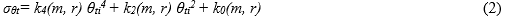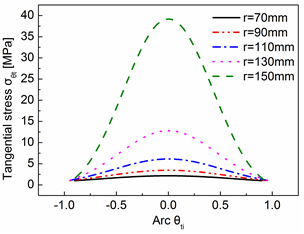Fig. 12. Tangential stress in the blade edge with respect to the polar coordinate system

The conditions used to determine k4(m, r)k2(m, r), and k0(m, r) were m values of 6, 7, 8, or 9 mm and r values of 70, 90, 110, 130, or 150 mm. The simulation results were shown in Figs. 13, 14, and 15.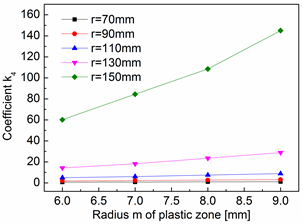Fig. 13. Variation of k4 with m at different r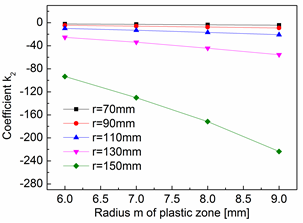Fig. 14. Variation of k2 with m at different r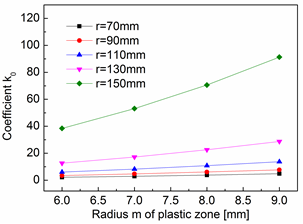Fig. 15. Variation curves of k0 with m at different r

The simulation results showed that k4k2, and k0 changed linearly with m and the slope changed at different r values. The mathematical models k4(m, r)k2(m, r), and k0(m, r) were built as follows, where f4(r)f2(r)f0(r)C4(r)C2(r), and C0(r) were related only to (Eq. set 3), based on the linear fitting method.

k4(m, r) = f4(r)(m – 6) + C4(r)

k2(m, r) = f2(r)(m – 6) + C2(r) (3)

k0(m, r) = f0(r)(m – 6) + C0(r)

Curves showing f4(r)f2(r)f0(r)C4(r)C2(r), and C0(r) as functions of r are shown in Figs. 16 and 17. Expressions of f4(r)f2(r)f0(r)C4(r)C2(r), and C0(r) were obtained using third-order polynomial functions by least-square fitting as shown below (Eq. set 4).

f4(r) = 1.955 x 10-4r3 – 0.056r2 + 5.246r – 160.194

f2(r) = -2.646 x 10-4r3 + 0.0756r2 – 7.009r + 213.288

f0(r) = 9.160 x 10-5r3 – 0.026r2 + 2.399r – 72.459 (4)

C4(r) = 3.618 x 10-4r3 – 0.102r2 + 9.450r – 290.106

C2(r) = -5.618 x 10-4r+ 0.145r2 – 13.523r + 410.296

C0(r) = 1.870 x 10-4r3 – 0.052r2 + 4.862r – 146.449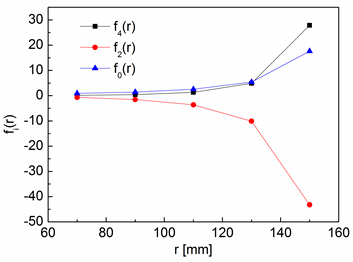Fig. 16. Variation of fi(r) with r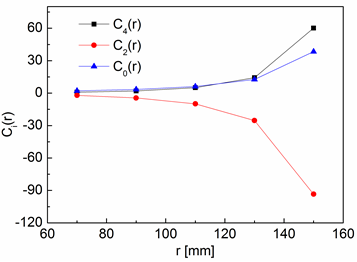Fig. 17. Variation of Ci(r) with r

Calculation model for the tangential tensioning stress of any point in the edge of circular saw blade

As shown in Fig. 18, the origin, O, of a rectangular coordinate system was located in the center of the circular saw blade. The coordinates of any point A at the edge of the circular saw blade were (R cos θ1, R sin θ1). The coordinates of any pressure spot, B, on the circular saw blade were (ri cos θ2i, ri sin θ2i). R was the radius of the circular saw blade, ri was the radius of the pressure spot, and di was the distance between points A and B (Eq. 5 and 6). A triangle was formed by points O, A, and B.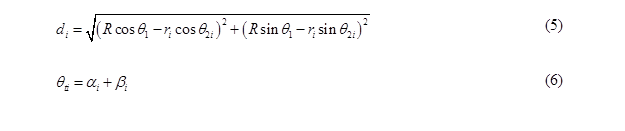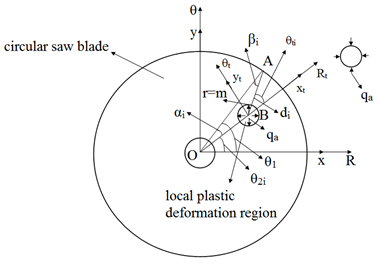Fig. 18. Calculation model for tangential tensioning stress in the edge of circular saw blade

Where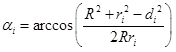and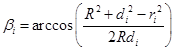. Eq. 2 was thus rewritten as Eq. 7,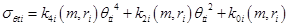(7)

Where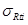and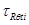were assumed to be 0. The stress was transformed as shown,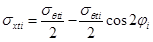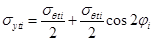(8)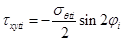When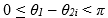or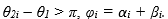When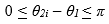or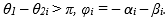The stress was again transformed as shown (Eq. set 9),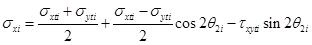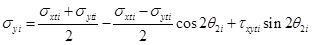(9)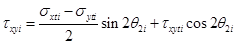The stress was transformed for the third time, as shown (Eq. 10),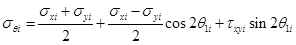(10)

Finally, the tangential tensioning stress of any point on the edge of the circular saw blade could be expressed by linear superposition as follows, where, i represents the first i pressure spots and numrepresents the total number of pressure spots (Eq. 11).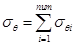(11)

RESULTS AND DISCUSSION

The theoretical values were close to the measured values, as shown in Table 1. The measured values were slightly less than the theoretical values because the measuring point was some distance away from the fixed point. The tangential tensioning stress of a, c, and e had the same values. The tangential tensioning stress of b and d had the same values. This showed that the distribution of tangential tensioning stress in the edge of the saw blade had regularity. The tangential tensioning stress in the edge of the circular saw blade was cyclical.

The mathematical model of the tangential tensioning stress on the edge of the circular saw blade tensioned by multi-spot pressure was shown to be correct. The tangential tensioning stress on the edge of the circular saw blade can be predicted accurately by the mathematical model.

Table 1. Contrast Between Theoretical and Measured Values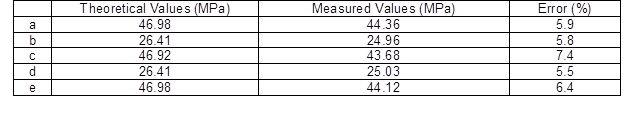CONCLUSIONS

1. In this study, a mathematical model for the tangential tensioning stress on the edge of a circular saw blade tensioned by multi-spot pressure was established by theoretical analysis and calculation. The model accurately predicts tangential tensioning stress on the edge of the blade and is very important for the quality control of circular saw blades.
2. The multi-spot pressure tensioning process of circular saw blades was assumed to include three kinds of mechanical processes: the one-spot pressure process, the elastic deformation of a disk with a through-hole subjected to uniform radial compressive stress, and the stress superposition process. The mathematical model for the tangential tensioning stress on the edge of the circular saw blade tensioned by multi-spot pressure was demonstrated as correct by the measured values.
3. The mathematical model for the tangential tensioning stress on the edge of the circular saw blade tensioned by multi-spot pressure establishes a theoretical foundation for the multi-spot pressure tensioning process of circular saw blades.

ACKNOWLEDGMENTS

We gratefully acknowledge the ﬁnancial support of the National Natural Science Foundation of China (No. 31270605) and the Agricultural Science Technology Achievement Transformation Fund of China (No. 2013G B24320607).

REFERENCES CITED

Carlin, J. F., Appl, F. C., and Bridwell, H. C. (1975). “Effects of tensioning on buckling and vibration of circular saw blades,” Journal of Engineering for Industry 97(1), 37-48.

Cristóvão, L., Ekevad, M., and Grönlund, A. (2012). “Natural frequencies of roll-tensioned circular saw blades: Effects of roller loads, number of grooves, and groove positions,” BioResources 7(2), 2209-2219. DOI: 10.15376/biores.7.2.2209-2219

Heisel, U., Stehle, T., and Ghassem, H. (2014). “A simulation model for analysis of roll tensioning of circular saw blade,” Advanced Materials Research 1018(2014), 57-66. DOI: 10.4028/www.scientific.net/AMR.1018.57

Ishihara, M., Noda, N., and Ootao, Y. (2010). “Analysis of dynamic characteristics of rotating circular saw subjected to thermal loading and tensioning,” Journal of Thermal Stresses 33(5), 501-517. DOI: 10.1080/01495731003659208

Ishihara, M., Murakami, H., and Ootao, Y. (2012). “Genetic algorithm optimization for tensioning in a rotating circular saw under a thermal load,” Journal of Thermal Stresses 35(12), 1057-1075. DOI: 10.1080/01495739.2012.720452

Nicoletti, N., Fendeleur, D., Nilly, L., and Renner, M. (1996). “Using finite elements to model circular saw roll tensioning,” Holzals Roh-und Werkstoff 54(2), 99-104. DOI: 10.1007/s001070050146

Schajer, G. S., and Kishimoto, K. J. (1996). “High-speed circular sawing using temporary tensioning,” Holz als Roh-und Werkstoff 54(6), 361-367. DOI: 10.1007/s001070050202

Schajer, G. S., and Mote, C. D., Jr. (1983). “Analysis of roll tensioning and its influence on circular saw stability,” Wood Science and Technology 17(4), 287-302. DOI: 10.1007/BF00349916

Schajer, G. S., and Mote, C. D., Jr. (1984). “Analysis of optimal roll tensioning for circular saw stability,” Wood and Fiber Science 16(3), 323-338.

Stakhiev, Y. M. (2004). “Coordination of saw blade tensioning with rotation speed: myth or reality,” Holz Roh Werkst 62(4), 313-315. DOI: 10.1007/s00107-004-0490-1

Szymani, R., and Mote Jr., C. D. (1974). “A review of residual stresses and tensioning in circular saws,” Wood Science and Technology 8(2), 148-161. DOI: 10.1007/BF00351369

Szymani, R., and Mote Jr., C. D. (1979). “Theoretical and experimental analysis of circular saw tensioning,” Wood Science and Technology 13(3), 211-237. DOI: 10.1007/BF00350225

Umetsu, J. (1989). “Confirmation of φ splitting in the distribution of residual stress in tensioning circular saws,” Journal of the Japan Wood Research Society 35(9), 856-858.

Umetsu, J., Noguchi, M., and Matsumoto, I. (1994). “Measuring residual stresses in tensioned circular saws using X-rays,” Journal of the Japan Wood Research Society 40(3), 268-273.

Article submitted: February 4, 2015; Peer review completed: April 19, 2015; Revisions accepted: April 29, 2015; Published: May 4, 2015.

DOI: 10.15376/biores.10.2.3798-3810